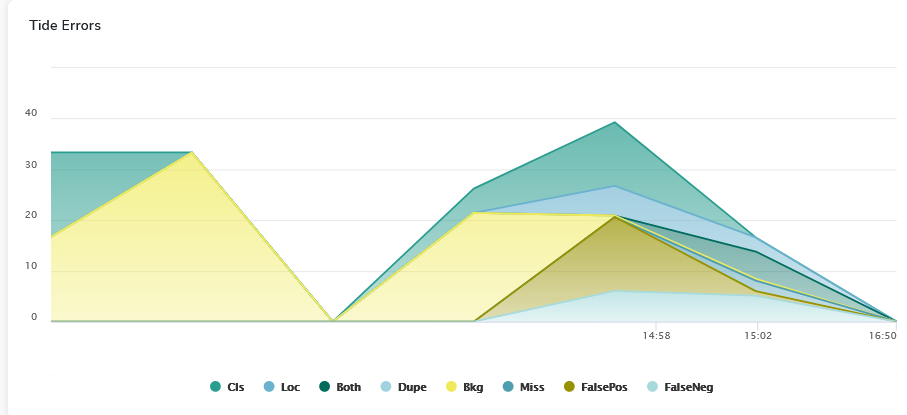# Tide Errors ( Object detection )

## Key Concept

It is interesting to understand where the recurrent errors of an object detection model come from so that its improvement is targeted.## Computation

Six types of recurring errors are defined using, for each prediction, thresholds on the IoU with the ground truth and the predicted class :

Classification Error (Cls) corresponds to a correctly localized prediction ($IoU{max} \geq t{f]$ ) but incorrectly classified.

Localization Error (Loc) corresponds to an incorrectly localized prediction ( $t{b} \leq IoU{max} \leq t_{f]$ ) and correctly classified.

Classification and localization error (Both) corresponds to an incorrectly localized and incorrectly classified prediction.

Duplicate Detection Error (Dupe) corresponds to the situation where the prediction is correctly localized and correctly classified but another correctly localized and correctly classified prediction exists with a higher confidence level.

Background Error (Bkgd) corresponds to a prediction that does not match any ground truth ($IoU{max} \leq t{b}$), i.e. the model has detected a part of the background.

Missed GT Error (Missed) corresponds to all undetected ground truth (false negatives) not already covered by classification or localization error.

The thresholds are set as follows : t{b} = 0.1 and t{f} = 0.5.

Since a false positive with a lower score has less effect on overall performance than one with a higher score, the errors are weighted so that they can be compared.